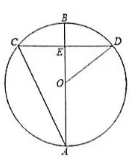$\text{A.}$ $\frac{5}{2}$ $\text{B.}$ $\frac{3}{2}$ $\text{C.}$ $\frac{1}{2}$ $\text{D.}$ $2$
【答案】 B